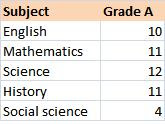# Statistics for Business Intelligence – Descriptive Statistics

In this post we look at descriptive statistics as a means for data exploration. Descriptive analysis refers to a group of methods that gives summary information about the data. For example consider the sales figures for a retail clothes outlet. An important figure would be the average of sales for a particular day of the week in a year.

Analysis of a single variable or Univariate analysis –
In most cases we need summary figures for a single variable, say height of students in a class or the maximum selling product during a sale etc. The methods in this analysis take as input various values for a single variable and provides summary statistics for it.

Types of summary statistics – There are mainly three kinds of summary statistics involved in univariate descriptive analysis.

1) Mean – This simly gives the average for all the values of the variable under consideration. for example if the marks scored by five students in a quiz are 6,8,9,5,8 then the mean is given by (sum of values)/(num of values)
or sum = (6+8+9+5+8)/5 = 7.2
The disadvantage of mean value is that an outlier can distort the mean to a very large extent.

2) Median – Median gives the central value in a group of values. In other words around half of the values are greater than the median and the other half are less than the median. consider the same number sequence as above.
6,8,9,5,8
arrange the sequence in ascending order
5,6,8,8,9
The central value is 8 and hence the median is 8. Median gives a number around which the values are distributed. If the series has an even number of observations then divide the middle two numbers by two to arrive at the median.

3) Mode – The mode is the value that is repeated the most number of times. In our series the mode is 8 since it is repeated twice.

The three summary values described above are labeled as measures of central tendency. In a normal distribution the values would be equal.

Another topic in Descriptive Statistics is Distribution. Consider a school that gives a grade to each student. A single variable distribution gives the number of students that have obtained Grade A for each subject.The same statistic can also be represented graphicallyThere are cases where the values of the variables are not discreet. consider a distribution of height for the students in the class. A distribution of each value of height vs number of students would probably give only one or two students for each height value. A better approach here would be to use a range of values instead of absolute values. In case of height use a distribution of this type:Dispersion
– Dispersion gives an idea of how the values are distributed around the central value. The measures of dispersion are range, mean absolute deviate, standard deviation and variance.

Range is the difference between the maximum and minimum value in a distribution. for example in the series 5,6,8,8,9 the range is 9-5 = 4.

Mean absolute deviation is an average of the absolute deviation of the numbers around the mean. The formula for mean absolute deviation isVariance – variance is the average of the squared deviations.Standard Deviation – It is the square root of variance. It has same units as the data used in analysis. Two methods are used to understand the significance of standard deviation. The first method is widely used and is applicable for all normal distributions (distributions that are symmetrical about the center and have a bell shaped curve). The method states that for normal distributions mean + one standard deviation(sigma) is equal to 68% i.e. 68% of the data are between mean+sigma and mean-sigma. 95% is between mean +- two sigma and 99.7% of the data are between mean+- 3sigma.
The second method is called ChebyChev’s theorem. It states that at least
1-1/square(k) of the values fall within +-k deviations from the mean. k > 1. for example 1-1/4 = 75% of values fall within +-2 deviations from the mean. The advantage of this theorem is that it can be applied to all distributions and not only normal distributions.

Note that the formula for variance and standard deviation described above are used for the population. To estimate values for a sample use a divisor of n-1 instead of n.

The last term for this section is coefficient of variation – it is the ratio of standard deviation to the mean expressed as percentage.
COV = standard deviation * 100/ mean